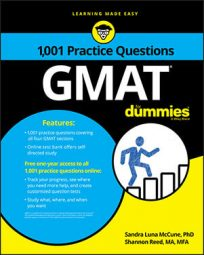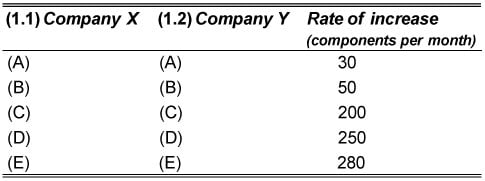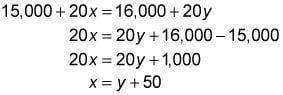##### GMATThe Two-Part Analysis questions on the GMAT present a short written explanation of a situation or math problem in which two portions of related information are unknown. You are asked to make two choices, one for each of the unknown portions.

Your answers for each of the multiple parts of an Integrated Reasoning question must all be correct to get credit for the question.

## Practice exercise

Use this information to answer the practice question.
Company X currently has 15,000 components in inventory, and company Y currently has 16,000 components in inventory. The number of components produced by company X and the number of components produced by company Y are increasing monthly, each at its own constant rate. Forecasters project that if each company continues to produce an increased number of components at its constant rate, in 20 months both companies will have the same number of components for the first time. Thereafter, in subsequent months, company X will have more components in inventory than company Y.
1. In the following table, indicate by appropriate selections in the first and second columns which of the rates of increase in the third column is a rate of increase for company X, and which is a rate of increase for company Y that together are consistent with the forecasters' performance projections.

Make only two selections, one in each column.1.1. The correct answer is D.

1.2. The correct answer is C.

Let x = the rate of increase for company X, and y = the rate of increase for company Y. Given that the number of components in inventory for the two companies will be equal in 20 months, set up an equation and solve for x in terms of y.This result tells you that company X's rate is 50 greater than company Y's rate. The only selections in the table where company X's rate is 50 greater than company Y's is 250 and 200, respectively.